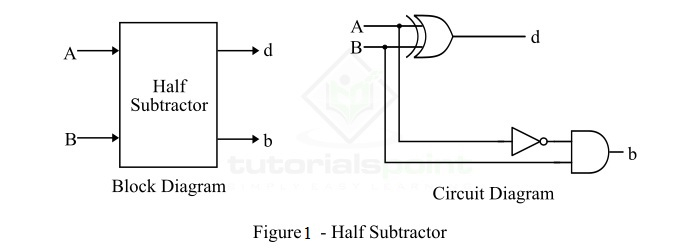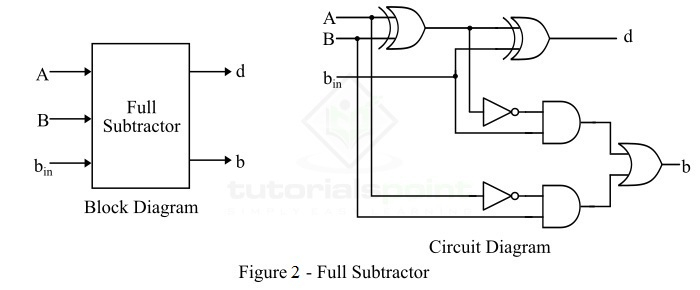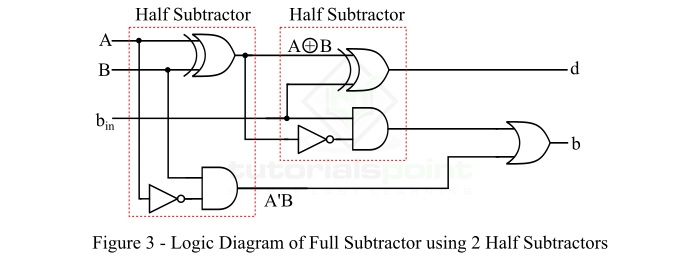# Realization of a Full Subtractor using Two Half Subtractors

A subtractor is a combinational logic circuit that can perform the subtraction of two numbers (or binary numbers) and produce the difference between them. It is a combinational logic circuit. Therefore, the output of the subtractor depends only on its present inputs.

There are two types of subtractors namely,

• Half Subtractor

• Full Subtractor

Read this tutorial to find out how you can realize a full subtractor using half subtractors. For the implementation of a full subtractor, we require two half subtractors. Let's start with a brief overview of half and full subtractors.

## What is a Half Subtractor?

A half-subtractor is a combinational logic circuit that has two inputs and two outputs where one output is difference bit (d) and another is the borrow bit (b). The half subtractor produces the difference between the two binary bits and also produces a borrow output (if any). In the subtraction (A-B), A is called Minuend and B is called Subtrahend bit.

The block diagram and logic circuit diagram of the half subtractor are shown in Figure-1.From the logic diagram of the half subtractor, it can be seen that a half subtractor can be realized using an XOR gate together with a NOT gate and an AND gate.

The difference bit (d) of the half subtractor is given by XORing the two inputs A and B. Therefore,

$$\mathbf{Difference,\:d=A\bigoplus B=A'B+AB'}$$

The borrow (b) of the half subtractor is the AND of A' (compliment of A) and B. Therefore,

$$\mathbf{Borrow\:,\:b=A'B}$$

## What is a Full Subtractor?

A full subtractor is also a combinational logic circuit which has three inputs A, B, bin and two outputs "d" and "b". Where, "A" is the minuend bit, "B" is the subtrahend bit, "bin" is borrow produced at the previous stage, d is the output difference bit and b is the output borrow bit.

The block diagram and circuit diagram of a full-subtractor is shown in Figure-2.From the logic diagram of the full subtractor, we can see that the implementation of a fullsubtractor requires two XOR gates, two NOT gates, two AND gates, and one OR gate.

Now, let us discuss the realization of full subtractor using two half subtractors.

## Implementation of a Full Subtractor using Two Half Subtractors

A full subtractor can be realized using two half subtractors. It will take two half-subtractors and one OR gate. The logic circuit diagram of the full subtractor using two half subtractors is shown in Figure-3.The first half subtractor performs XOR operation on input bits A and B, and AND operation on A' and B to produce an intermediate borrow bit

The second half subtractor performs the XOR operation on the output of first XOR gate and the input borrow bit $(b_{in})$, and the AND gate of the second half circuit gives an output equal to (A'B+AB')'.$b_{in}.$

The output of the second XOR gate is the output different bit (d), and the output borrow bit (b) is obtained by ORing the outputs of two AND gates.

In this way, we can realize a full subtractor by cascading two half subtractors, as shown in the above figure